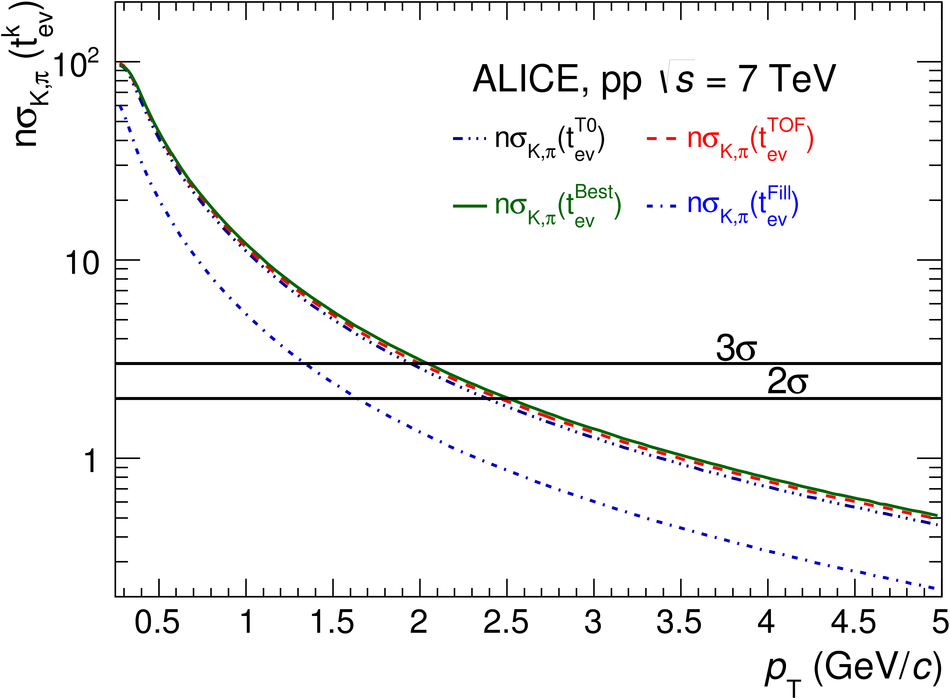# Determination of the event collision time with the ALICE detector at the LHC

Particle identification is an important feature of the ALICE detector at the LHC. In particular, for particle identification via the time-of-flight technique, the precise determination of the event collision time represents an important ingredient of the quality of the measurement. In this paper, the different methods used for such a measurement in ALICE by means of the T0 and the TOF detectors are reviewed. Efficiencies, resolution and the improvement of the particle identification separation power of the methods used are presented for the different LHC colliding systems (pp , p-Pb and Pb-Pb) during the first period of data taking of LHC (Run 1).

Figures

## Figure 1

 A schematic layout of one of the 18 TOF supermodules inside the ALICE spaceframe.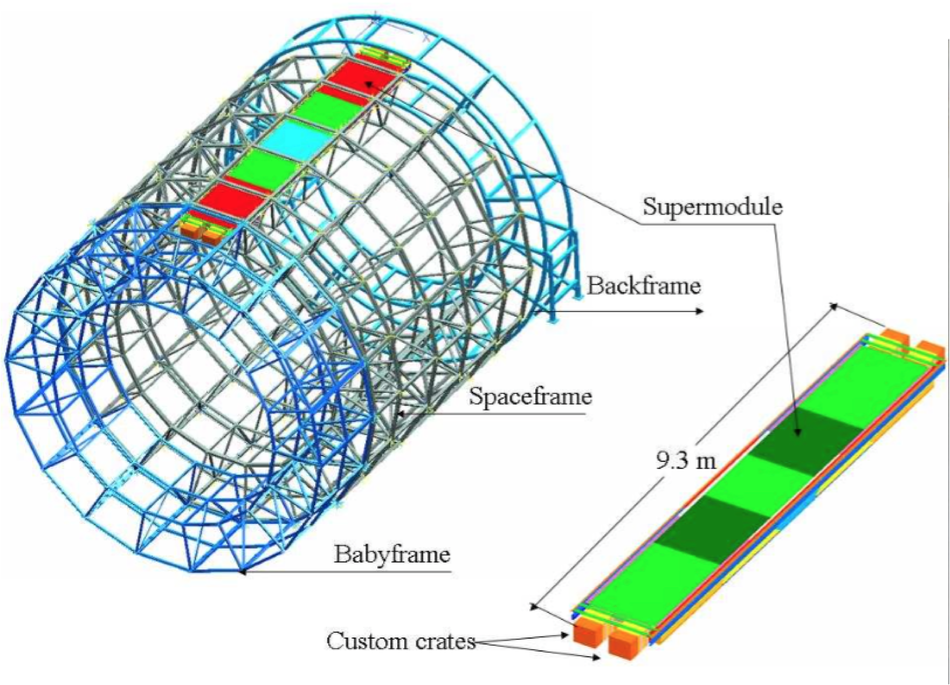## Figure 2

 The layout of the T0 detector arrays inside ALICE.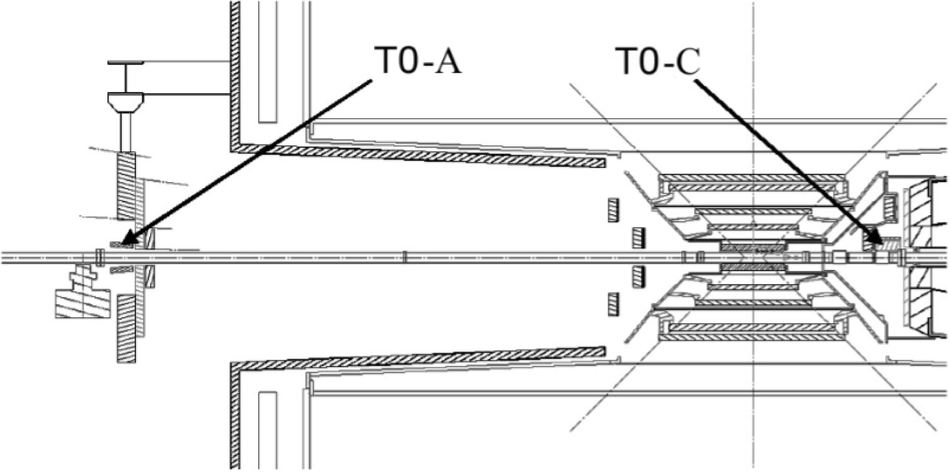## Figure 3

 Average collision time $\langle{t_{\rm ev}}\rangle$ calculated for five minutes of p-Pb data taken at $\sqrt{s_{\rm NN}}$= 5.02 TeV.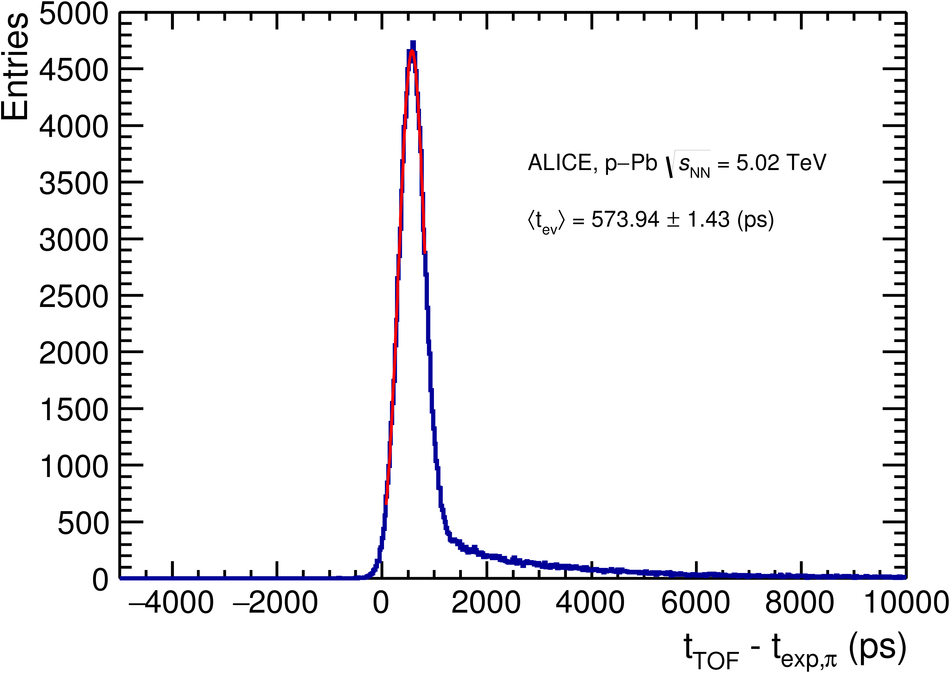## Figure 4

 Resolution of the $t_{\rm{ev}}^{\rm{Fill}}$ ($\sigma_{t_{\rm{ev}}^{\rm{Fill}}}$) for all 335 pp runs recorded at $\sqrt{s}$= 7 TeV in 2010.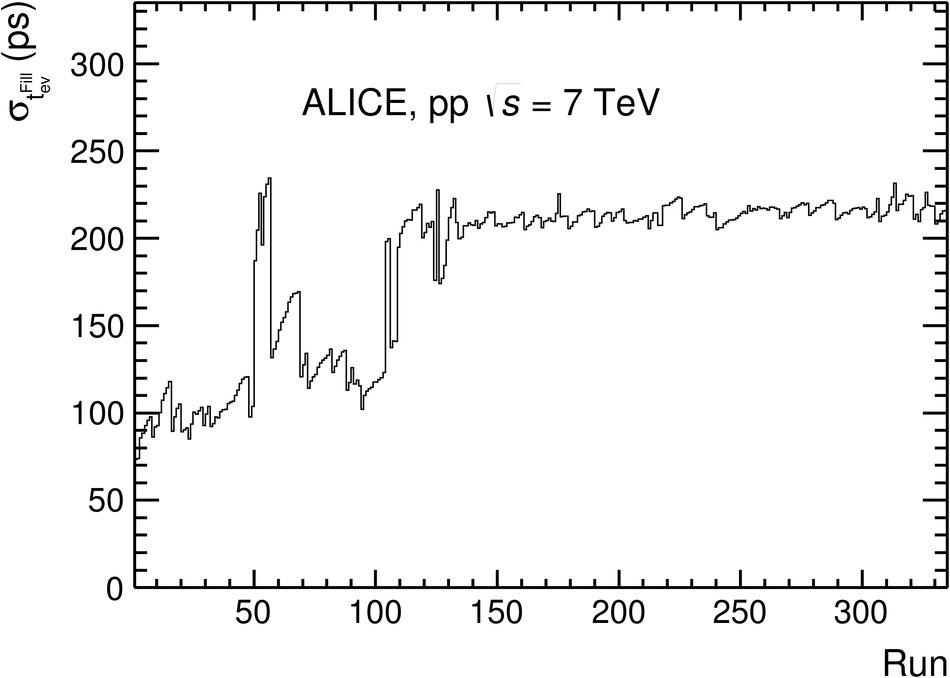## Figure 5

 Efficiency of the $t_{\rm{ev}}^{\rm{T0}}$ (circles), $t_{\rm{ev}}^{\rm{TOF}}$ (squares) and $t_{\rm{ev}}^{\rm{Best}}$ (diamond) as a function of the TOF track multiplicity for pp collisions at $\sqrt{s}$= 7 TeV.## Figure 6

 Efficiencies of the methods $t_{\rm{ev}}^{\rm{T0}}$ (circles), $t_{\rm{ev}}^{\rm{TOF}}$ (squares) and $t_{\rm{ev}}^{\rm{Best}}$ (diamond) as a function of the V0A multiplicity class for p-Pb collisions at $\sqrt{s}$= 2.76 TeV (top) and of the V0M Centrality class for Pb-Pb collisions $\sqrt{s_{\rm NN}}$= 2.76 TeV (bottom).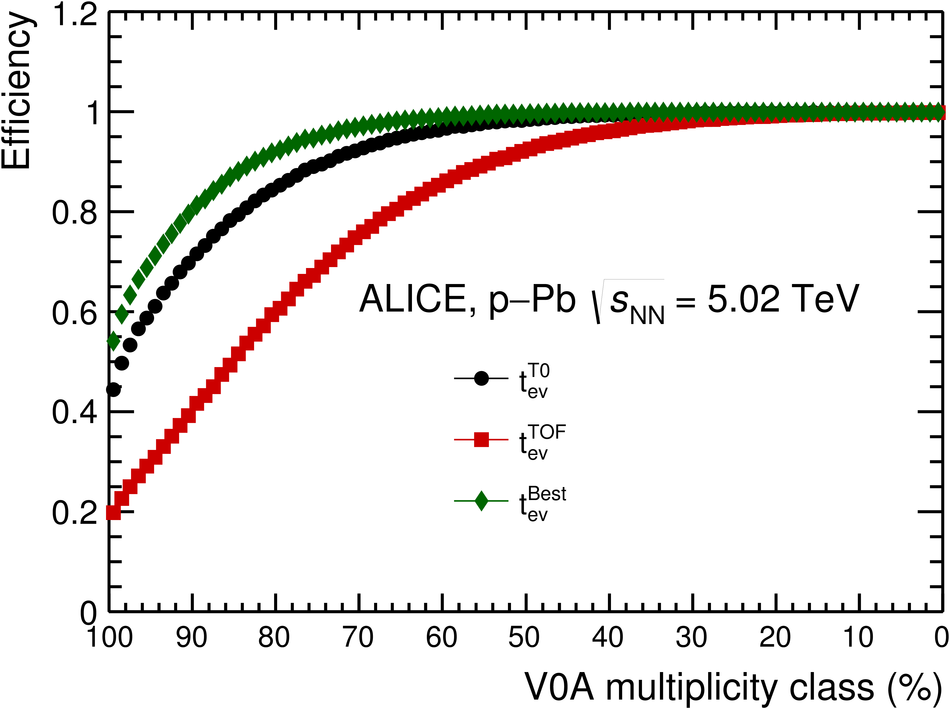## Figure 7

 Resolution of $t_{\rm{ev}}^{\rm{TOF}}$ (top) and $t_{\rm{ev}}^{\rm{Best}}$ (bottom) as a function of the TOF track multiplicity for pp collisions at $\sqrt{s}$= 7 TeV (star), p-Pb collisions at $\sqrt{s_{\rm NN}}$= 5.02 TeV (circle) and Pb-Pb collisions at $\sqrt{s_{\rm NN}}$= 2.76 TeV (square). As a reference, TOF track multiplicity=15 corresponds to 50% V0A multiplicity class in p-Pb and 80% centrality class in Pb-Pb.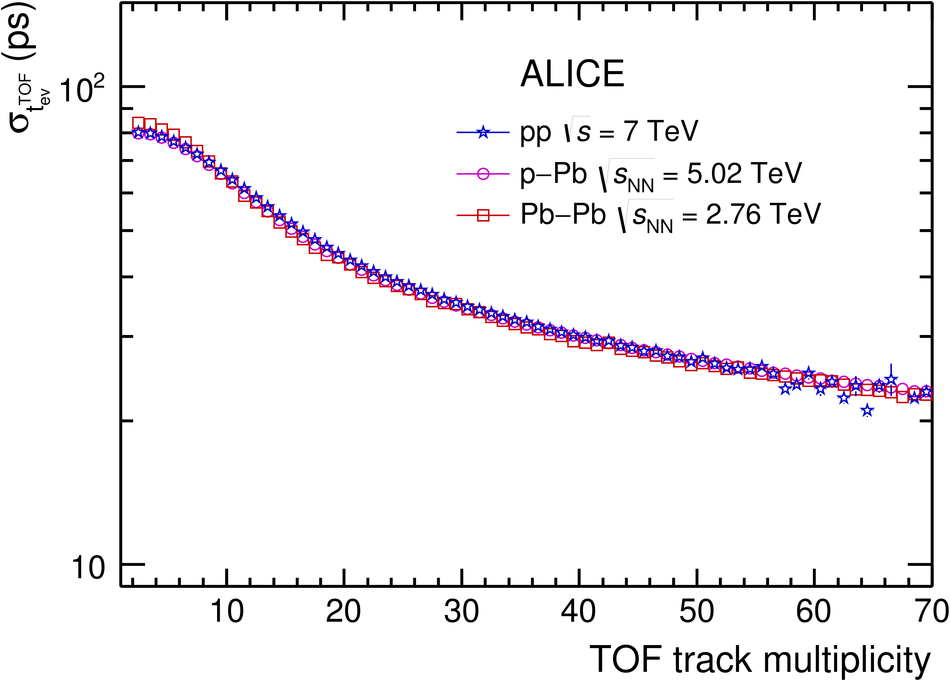## Figure 8

 Fraction of events for which the $t_{\rm{ev}}^{\rm{Best}}$ is provided exclusively by the T0, no matter if T0A, T0C or T0AC (circle), or exclusively by the TOF (square) or a combination of the two (green) in Pb-Pb collisions at $\sqrt{s_{\rm NN}}$= 2.76 TeV.## Figure 9

 K-$\pi$ (top) and p-K (bottom) separation power as a functionof the transverse momentum of a track when $t_{\rm{ev}}^{\rm{TOF}}$ (dashed line), $t_{\rm{ev}}^{\rm{T0}}$ (dash-dottet line), $t_{\rm{ev}}^{\rm{Best}}$ (solid line) and $t_{\rm{ev}}^{\rm{Fill}}$ (dotted line) are used.